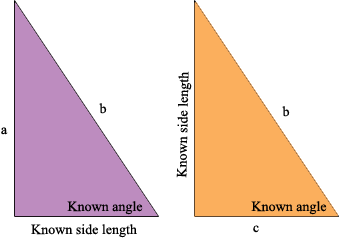SEARCH HOMEMath Central Quandaries & QueriesQuestion from ayesha, a student: how to find the other two sides of right angle triangle when length of one side and angle of other side is given i.e 45Ayesha,

You have one of these two situationsFor the triangle on the left write down the three expressions

sin(Known angle)
cos(Known angle)
tan(Known angle).

One of these three expressions will contain Known angle, Known side length and a and another will contain Known angle, Known side length and b. Solve for a and b.

Use a similar technique for the triangle on the right.

PennyMath Central is supported by the University of Regina and The Pacific Institute for the Mathematical Sciences.